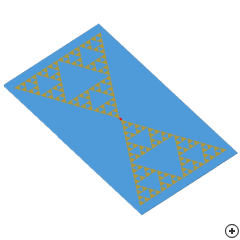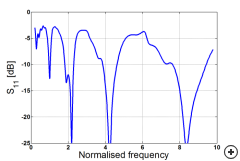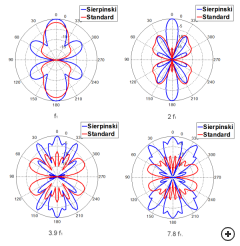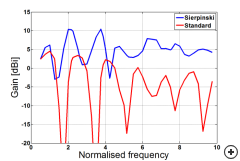Image of the Sierpinski gasket antenna.

The Sierpinski gasket is a simple fractal geometry that provides multi-band performance in antenna applications. This antenna's bands are typically spaced factor 2 apart (as shown in a typical s11 vs frequency plot below - the first 3 operating bands are shown here). By adjusting the fractal geometry, other band spacings are can be achieved.Typical reflection coefficient vs frequency (normalised by the first iteration fractal operation band frequency f1) with reference impedance of 100 Ohm.

The Sierpinski gasket is a simple fractal geometry that provides multi-band performance in antenna applications. This antenna's bands are typically spaced factor 2 apart (as shown in a typical s11 vs frequency plot below - the first 3 operating bands are shown here). By adjusting the fractal geometry, other band spacings are can be achieved.

The antenna's pattern and impedance performance in each band are identical, up to the point where truncation and substrate effects come into play. This makes the Sierpinksi bowtie suitable for applications where matched multi-band performance is important.

Physically the Sierpinski bowtie is quite similar to the standard bowtie and similar construction techniques may be used. Although the Sierpinski bow tie is more complex to manufacture when compared to a standard bowtie antenna, its impedance and 3D pattern are much more stable across band. The images below compare typical gain performance between the Sierpinski gasket and a standard bow tie with the same outer dimensions at various frequencies. The last image shows comparison of typical boresight gain (theta=0, phi=90) vs frequency for both these antennas.Comparison of typical normalised gain (phi=90) in dB vs. Angle for Sierpinski and standard bow-tie with the same outer dimensions at 1x, 2x, 3.9x and 7.8x f1.Comparison of typical boresight gain (theta=0, phi=90) vs frequency (normalised by the first iteration fractal operation band frequency f1) in dBi for a Sierpinski gasket and standard bow-tie of the same outer dimensions.